Grid Overview

A grid proposal consists of several contiguous, or nearly contiguous, observations. They may also sometimes be refered to as "raster" proposals.

The term "grid" or "raster" is slightly misleading in that:

• The region can be irregular,
• The pointings will not "line up" in a grid unless a roll or group constraint is applied.

An idealized survey layout is depicted below. This survey consists of nine ACIS-I pointings in a 3x3 grid.

Figure 1: ACIS-I Grid Pointing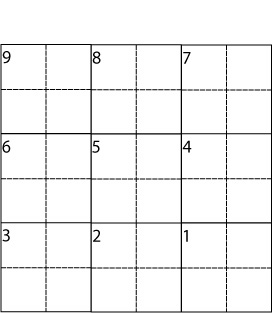Figure 1: ACIS-I Grid Pointing

A schematic figure showing the layout of a 3x3 ACIS-I grid proposal.

Slew Tax for Grids

A "slew tax" of 1.5 ks is added to each observation at the Chandra Peer Review. This represents the average slew, settle and set-up time required for an observation.

Because some or all of the pointings in a grid can often be done consecutively, the average slew time is less than the nominal 1.5 ks and is calculated differently. To qualify for the reduced slew tax, the observations in a grid must satisfy the following conditions:

• Maneuvers from one observation to the next must be less than or equal to one degree
• There can be no change in instrument configuration.
• Individual exposure times must be 44 ks or less

To calculate the reduced slew tax:

• Pointings will be assembled into one or more groups with a maximum of 90 ks per group, including slew tax.
• The slew tax for the first observation in a group will be 1.5 ks. Subsequent observations in the same group are charged at a rate of 0.5 ks.

Programs which qualify for reduced slew tax also qualify for a reduced "time constrained count." Time constrained observations impact Chandra's observing efficiency. Short, closely spaced observations which can be scheduled as a group have less of an impact on Chandra's efficiency than the same observations spread over the sky.

Note: a "group" for the purposes of determining slew are not the same as proposer-requested groups.

Consider a survey with 20 pointings each of 5 ks.

• Number of pointings: 20
• Expo per pointing: 5
• Each group is as close to 90ks as possible
• Group 1
• 1 (5ks obs + 1.5ks of slew)
• 15 (5ks obs + 0.5ks of slew)
• Total = 80ks obs + 9ks slew = 89 ks
• Group 2
• 1 (5ks obs + 1.5ks of slew)
• 3 (5ks obs + 0.5ks of slew)
• Total = 20ks obs + 3ks slew = 23 ks
• Over the whole proposal:
• Number of groups: 2
• Total exposure: 100ks
• Total slew tax: 12ks

Please note that grid programs are unconstrained unless the proposer specifically requests a constraint. If the proposer requests a constraint, the groups for calculating the constraint values are defined the same as for slew tax calculations.

Roll and Grouping Constraints

The observations in a grid are NOT automatically constrained.

In practical terms, this means that that they may scheduled at any time during the cycle. Since the nominal roll of Chandra changes with time, the roll angle of each observation may be different.

If a proposer does not request any constraints, THE ROLL ANGLES WILL NOT LINE UP, as illustrated in the following figure. This will most likely lead to gaps in the sky coverage.

Figure 2: Unconstrained Grid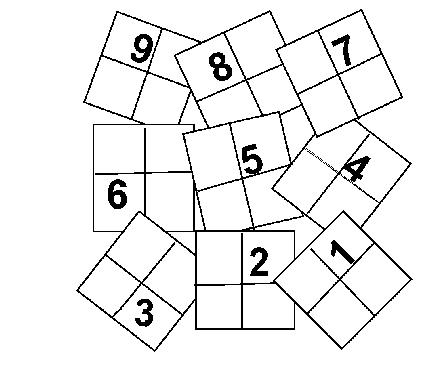Figure 2: Unconstrained Grid

What a grid observation may look like without a roll constraint.

It is often most efficient to place several pointings from a survey contiguously in the observing schedule (this minimizes slew time). The number of such pointings that can be accommodated before slewing to a different location on the sky is dependent on the pitch angle of the survey observations.

The most likely outcome for a survey with many short pointings is for a group of them to be scheduled together, followed by additional groups at later dates, until the observations are complete.

Observations within such a group will have essentially the same roll, but different groups will have different rolls.

If it is essential to have uniform coverage, the proposer must restrict the roll angles of the observations. This can be done in two ways:

• Impose a roll constraint (with associated tolerances) if the roll angle of the survey must be fixed.
• A group constraint should be used if the absolute value of the group constraint forces all the observations to be done within a certain time interval, but does not specify the exact window (e.g. all done within 30 consecutive days, but the 30 day period can start at any time during the cycle).

Determine Roll Tolerance

The example illustration consists of nine 70 ks pointings in a 3x3 grid covering 0.6 square degrees where the absolute value of the roll is not important. It is important not to have gaps in the coverage. This is a case where a group constraint should be used, instead of a specific roll constraint.

The maximum acceptable change in roll between two adjacent pointings is illustrated in the following figures.

Figure 3: Zero Roll Angle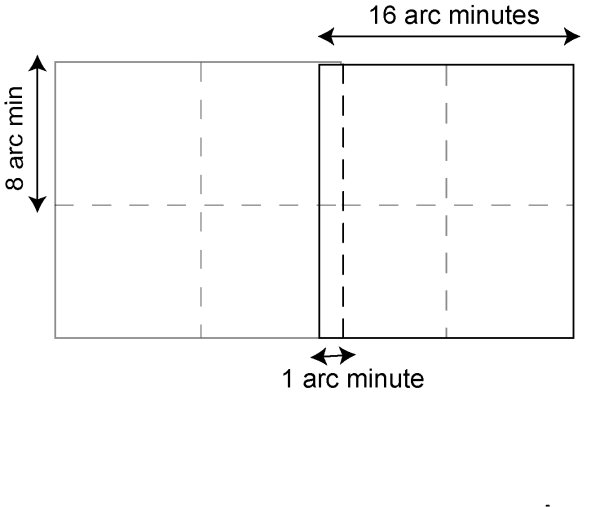Figure 3: Zero Roll Angle

Two adjacent fields at zero roll angle. Note the overlap between the two chips.

Figure 4: Unacceptably Large Roll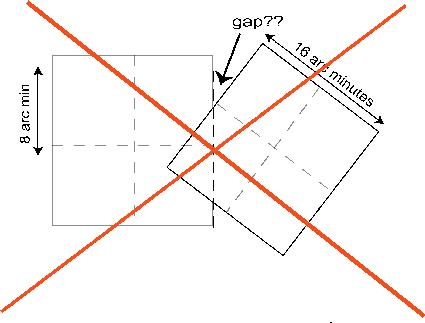Figure 4: Unacceptably Large Roll

Two adjacent fields where the second field is rotated: this is NOT acceptable for our example because there is a gap.

Figure 5: Maximum Roll Difference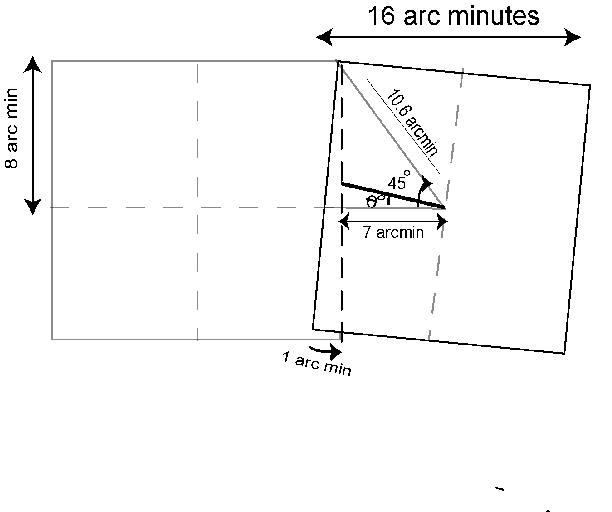Figure 5: Maximum Roll Difference

Two adjacent fields with maximum roll difference. Further rotation will create a gap. This geometry is correct if the overlap between two adjacent chips is small compared to the width of the chips (in this case the overlap is 1 arcmin and the ACIS-I chips are 16 arcmin on a side).

The maximum roll (theta) is calculated from:

cos(45 - theta) = 8/10.6
45 - theta = 41
theta = 4 degrees

Evaluate Group Constraint

The roll angle may change by a maximum of +/-4 degrees between the first and last pointing. The corresponding time interval can be estimated using ProVis tool. The resulting plot contains roll and pitch angles and target visibility as a function of time. Inspection of the plot can give a reasonable estimate for the rate of change of roll with time. Say in this example, that is about 7 degrees per week. Therefore, +/-4 degrees tolerance translates to about 8 days. The RPS group parameters would then be set as follows:

• Set Group Observation Flag to Y
• Enter "Group Identification" (can be any string to identify targets)
• Time Interval for Group=8 days

Number and Type of Constrained Observations

Both Constraints and Slew Tax can be obtained with the "Constraints/Slew Tax" button in RPS once forms have been filled out. Keep reading for an example constraint calculation made without the RPS tool.

Constrained observations are classified as "Easy", "Average" or "Difficult" (see the Call for Proposals). The dimensionless grading parameter for a group constraint is as follows:

```
Time Interval for the Group
---------------------------------------
Total Duration of Observations in the Group
```

The time interval for the example group is 8 days. The total duration of the observations is 70x9 = 630 ks or 7.3 days. The grading parameter then is 1.1. According to Table 5.1 of the Call for Proposals a group grade of 1.1 is considered "difficult". This particular example, which contains 9 observations, therefore counts as 9 Difficult observations.

Please note that if the exposure time for each observation was 44 ks or less, the number of time constrained observations would be charged at a reduced rate, as described above.

RPS Grid Parameters

It is possible to specify a grid in one of two ways:

• Enter each position as a separate target, or
• Use the grid parameters. These are:
• Coordinates of survey center
• Number of pointings
• Distance from the survey center to the farthest grid pointing
• Total exposure time (sum of all pointings)

In the second scenario, CXC staff will contact PIs of successful grid proposals after the Peer Review to request their required list of target positions.